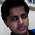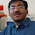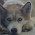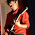### Lion in a Circular Cage Puzzle

Source:
Asked to me by Pramod Ganapathi (PhD Student at Stony Brook University)

Problem:
A lion and a lion tamer are enclosed within a circular cage. If they move at the same speed but are both restricted by the cage, can the lion catch the lion tamer? (Represent the cage by a circle, and the lion and lion tamer as two point masses within it.)

1.Seems to be. Let v1 be the tamer's speed away from lion and v2 be the speend of lion towards the tamer. The lion can always choose to maximise this speed by directing himself towards tamer. But, for tamer to always be with same speed, (which is maximum of his speed as well), both will have to run in same direction all the time. Since this is not possible in the restricted area, lion will catch tamer eventually (Assuming intelligent lion :))

2.By the way, best strategy for tamer to avoid getting caught for longest time, is to stay close to lion and run in circles. :)

3.Lion would definitely catch the tamer. The lion can start from Center of the cage and then tamer would be at some point on the circumference(to be furthest from lion). Then tamer can move only along the circumference while loin would move towards him. Since both are moving with same speed, hence the distance bw lion and tamer would keep decreasing and lion would eventually catch the tamer.

4.As I see it the distance between them can only decrease. Hence yes.

5.I think the problem is more accurately re-stated that is there a pattern of movement by the Tamer such that the Tamer escapes the lion.

Because obviously if both the tamer and lion move at the same speed towards each other the lion will catch the tamer.

My attempt:

Maybe one way to envisage is that no matter what the location of the trainer, he can move at least as far away from the lion as the lion moves to the trainer by backing up towards the edge of the ring.

Therefore we are in the configuration where the tamer is on the edge and the lion is an arbitrary distance away.

We can further reduce the problem down because if the trainer can escape whilst the lion is infinitesimally close then the trainer can just "wait" until the lion gets that close and then move.

Lets consider the case where a the lion is y distance away from the trainer and the trainer at most can move perpendicular to the lion . (in the local neighbourhood the cage edge is flat, and at right angles to the lion-trainer.

If in this scenario the lion moves in any direction other than immediately towards the trainer, the trainer can increase the distance between them by going the other way around the cage.

The furthest the trainer can move per dt, is dx away from the lion, along the cage wall, and the closest the lion can move is dx towards the trainer.

===============
<--x---T----x-->
|
|
y
|
|
L

The distance between the trainer and lion is therefore

( dx^2 + (y - dx)^2) ^0.5, the Lion catches the trainer if this equals 0. therefore The lion can catch the tamer for some speed if the equation

dx^2 + y^2 - 2 y dx + dx^2 = 0

2dx^2 - 2 y dx + y^2 = 0

has real roots if (-2y)^2 - 4 * 2 y ^2 > 0 for all values of y.

i..e if 4y^2 - 8 y ^2 > 0

i.e. if -4 y ^2 >0 .

Which is not true as y is a real number.

Therefore the distance between lion and tamer does not have real roots, and as such the lion can not catch the tamer. for any speed, for any arbitrary >0 starting distance from the tamer.

6.7.Is there any penalty for changing direction?

8.Haven't thought it through completely but my gut says yes.

If the lion moves to the center of the cage the tamer will have to move an equal or greater distance to get away from the lion than the lion does to block his passage.

9.If the question is "Is there a strategy for the lion to certainly eat the tamer ?", there one (well, it can come as close as he wants, the last epsilon is trickier).

First, it should go the center. Then, it should go directly toward the tamer. Since it is closer to the center than the tamer, it has a greater orthoradial speed than him. Therefore, whatever the trajectory of the tamer, the lion can be on the same radius. But since, they are supposed to run at the same speed, the lion can use the remaining part of its speed to close in on the tamer.

Eventually, it will get as close as it wants (but not on him). Then it depends on what the tamer does. If he never keep running along the cage, he will be safe but infinitely close to the lion... :) Maybe it will be able to paw to close the epsilon distance...

10.I see two possibilities. Either the lion can always catch the tamer, or the tamer can get away from the lion moving on the border of the circle. My thinking goes like this : First, the lion doesn't care what tamer does and runs to the center of the circle. Then, lion moves in such a way, to always stay on one radius with the tamer. Since the lion is closer to the center of the circle than the tamer, he doesn't have to use all of his speed to just stay on the radius, he can also shorten the distance between himself and the tamer on the radius by a little bit. The question is reduced to calculate whether the amount by which he gets closer to the tamer is enough, to catch him when he runs on border of the circle. I'll try to do some calculations (probably invovling polar coordinates), and post if I'll come up with something.

11.My argument uses two primary assumptions

-In the general case, the tamer wants to get to be the furthest distance it can from the lion.

-As an end goal, the lion wants to reduce that distance with every move.

If the two starting positions for the tamer and the lion are on the furthest points of the diameter of the circular cage, the lion can reduce the distance to the tamer with every move, as the only move possible for the tamer following that algorithm is to go towards the lion.

In reaction to this, the tamer would make a beeline at a tangent to the circle, but the lion can reduce distance by calculating the next move it needs to make to remain on the radius of the circle whilst reducing that distance to 0.

Applied to the more general case, all the lion needs to do is move into the radial position outlined above, and repeat the algorithm. Factoring in randomness means that this is a little more problematic, but it still provides the possibility.

So yes, there is a possibility that, given a circular cage, a lion could catch a tamer when applied to a mathematical model.

12.Seems like an easy problem to me. No matter where you place the lion and the tamer in the circle, the lion always has the advantage that it can move to the centre of the circle (assuming the lion tamer is trying to keep as far away from the lion as possible). Once in this position, the lion travels a shorter distance to the outside of the circle (even if following an arc), than the lion tamer will travel on the outside of the circle). I'm sure you can work out a mathematical proof for this if you wish.

13.Yes, the lion will catch the tamer.

At any instant, let V be the vector from the lion to the tamer.

The lion’s pursuit algorithm is the simplest one: move toward the tamer (along V).

The tamer has a choice: either run along V (away from the lion) or along some other vector, U. Except when the tamer is at the fence, in which case V is not available. At any instance that the tamer is not running along V, the lion is shrinking the distance to the tamer, for the same reason that a bicycle’s rear wheel covers less distance than the front wheel (intuitively, the lion is able to cut corners).

All we need to show now is that the convergence takes finitely long. The only way that convergence can take forever is if the tamer has a way to slow down the rate at which the lion is gaining on him; for example by running along an infinite straight line, or in an outward spiral whose curvature steadily approaches a straight line. That cannot be, because the curvature of any sufficiently long path is constrained by the fence which is finite in radius.

14.15.Just building up on Michael's solution :-

Let both their speeds be v. And without loss of generality we can assume lion is in center of circle and tamer is on an edge.

(Lion can come to this position anytime, no matter what the tamer does)

Let the speed of lion and tamer (which are equal) be v

Consider this vector diagram

^
|
lion -> tamer

For the lion to catch the tamer, it has to come on the hypotenuse of this diagram. If it can come in root(2)*v it can catch the tamer in one go. But it can't. So, for the time being it has only reduced the distance between the tamer and itself.

Now for convergence of this series, the argument is same as that of Michael. Since the curve is convex inside, the tamer cannot keep on avoiding the lion for infinite time.

So the lion will catch the tamer.

16.Point 1 : Though i can tell a man to run in a circle , How do i tell a lion to do the same thing.... :P
Unless he is tamed :D

In case he is tamed , Why will he eat up his tamer satisfy his hunger for a while and get shot for being a man eater. Rathen then enjoy delicious mutton chops the tamer will bring him everyday.

In case he is not tamed , Why is the tamer in this profession , if not to tame The Lion.

I think eventually Tamer will catch the Lion or better he can climb the cage to prevent any other consequence ... :P

17.Yup,
All scenarios are possible.
Sorry to break the fun but this is the first problem in "The art of mathematics" by Bela Bollobas. I have a good habit of atleast remembering the first problem of the good books I have read.

18.I think that the lion will be able to catch the tamer. My strategy for the lion goes like this.
The lion first moves to the centre of the circle.
Now,
Claim 1: The lion can always move in such a manner when its distance from the tamer is not increasing (it can move in the parallel direction)
Now, the tamer can not follow a direction indefinitely as its arena is bounded by a circle. So, he will change the direction many times.
Claim 2: Let X be the position of the tamer and Y be the position of the lion. Join XY. Take a line perpendicular to XY, say L. Now, tamer can either move on L, or away from lion on other side of L, or in a direction to the same side of L as the lion. In the first two cases, the lion can always move to let his distance remain constant. In the third case, the lion can move to reduce the distance.

Now, the lion will move in the manner given in Claim 2 and the tamer will eventually have to move acc to case 3 many times and the lion will eventually catch it.

19.Lion will be able to catch the tamer asymptotically (in infinite time!!!). Let's assume the lion starts from the center and tamer is on the boundary (any other scenario can be reduced to this one). Now lion will run so that his angular velocity is the same as that of tamer and both are on the same radius. Now since the lion is always running on a smaller circle than tamer (an intelligent tamer will always run on the outermost circle), lion always has an extra speed component which it can use to get radially close to the tamer. Lion's trajectory will be spiraling outwards. But, this expendable speed component will keep on reducing as lion gets closer (because more speed will be needed to maintain the angular part). So, since both are point masses, lion won't be able to actually catch the tamer.

20.Lion will catch the tamer.
Let lion and tamer be on opposite side of diameter (farthest distance). Now both move in a particular direction along the circumference. Since speed of both lion and tamer are the same, they will always be on the opposite ends of a diameter.
Now, let the lion move towards the center by dx. The tamer is moving along the circle of radius r. And the lion is moving along a circle of radius r-dx. Hence lion will be able to complete the circle faster than tamer. So, a time will come when both the lion and tamer will be on same radius line (separated by distance dx). dx->0 implies the tamer will be caught.

21.Very strangely I feel lion may not catch the tamer. I have an assumption that the tamer will make an intelligent move so as not to be caught. Let d be the initial distance between them in the circuar cage. Assume d is represented by a hard stick. Assume the centre point of d is C. In a frame of reference located at C and at which is moving at a velocity ( V1 + V2 )/2 , the distance between the tamer and the lion can be kept a constant = d.

22.I remember struggling with this problem a lot but could not resolve it!!
The answer is NO, the man can always escape.
It was proposed in 1930's and proved after 2 decades by Besicovitch, his solution is given in
JE Littlewood's - A Mathematicians Miscellany(Page 143).
His basic idea is to construct a infinite length polygonal path all along which the lion cant catch the man. First lets try to counter the trivial first solution which comes up to our mind - The case where the lion always moves along OM (O is the center, M is the mans position). So take this path M_nM_n+1 is perpendicular to OM_n, let |M_nM_n+1| = L_n. Now we have |OM_n|^2 = |OM_n-1|^2 + |M_n-1M_n|^2. thus |OM_n|^2 = |OM_0|^2 + L_n^2 + L_n-1^2+....+L_1^2. So we now choose {L_i} in such a way that sum{L_i^2} < infty but sum{L_i} = infty. Also note that when M is moving along M_0M_1 and L along OM, L cant catch M as say if the meet at N then we have a contradiction as |ON| > |M_0N|(hypotenuse > side) also length traversed by lion > ON (st line between 2 points is the shortest among all the curves). Thus L cant catch M while on M_0M_1, similar argument shows that L can never catch M on any of the segments, thus a contradiction.
Now he extends this to any strategy that L can follow so basically at M_n let L be at P_n then contruct M_nM_n+1 perpendicular to P_nM_n and let N_n be the foot of the perpendicular from O onto M_nM_n+1 now we choose |N_nM_n+1| = L_n(M_n+1 and M_n are on opposite sides of N_n, {L_i} the divergent series which is square summable), now we have |OM_n|^2 - |OM_n-1|^2 <= L_n^2, so even this path lies in the circle. As |M_nM_n+1| > |N_nM_n+1| = L_n this path has infinite length, and according to the previous argument L cant catch M all through this path.

23.Assumptions: Lion starts at the center, trainer at the edge. Trainer runs along the edge with velocity v. Lion keeps on the same radius as the trainer and heads towards the trainer also so as to keep its velocity equal to v as well. The radius of the cage is R.

By my calculations the lion catches the trainer in finite time (Pi R)/(2 v).

24.Assumptions: Man at edge runs along the edge with velocity v. Lion starts and center (0,0) and runs towards the mans so as to keep on the same radius as the man and get closer with its velocity equal to v also. The radius of the case is R. Man starts at (R, 0) and runs counterclockwise.

Position of man at time t is (R cos(vt/R), R sin(vt/R)).

Position of lion at time t is (r cos(vt/R), r sin(vt/R)).

Take the derivative with respect to t to get the velocity vector. Set norm of velocity vector to v to get: v^2=(r')^2+(rv/R)^2.

Solve this ODE to get r=R sqrt(tan(vt/R)/(1 + tan(vt/R)).

When vt/R=Pi/2 we will get r=R and the lion reaches the trainer.

The lion gets to the trainer in finite time: (pi R)/(2 v)

25.the same question was asked in its modified form in IIT KANPUR in an event INSTANT as follows -

"find the maximum time it will take lion to catch the men. "